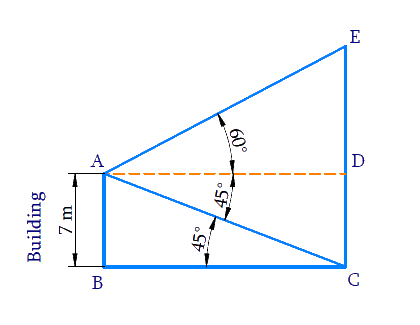# Ex.9.1 Q12 Some Applications of Trigonometry Solution - NCERT Maths Class 10

Go back to  'Ex.9.1'

## Question

From the top of a $$7 \,\rm{m}$$ high building, the angle of elevation of the top of a cable tower is $$60^\circ$$ and the angle of depression of its foot is $$45^\circ$$. Determine the height of the tower.

Video Solution
Some Applications Of Trigonometry
Ex 9.1 | Question 12

## Text Solution

What is Known?

(i) Height of building $$=7 \,\rm{m}$$

(ii) Angle of elevation of the top of a cable tower from building top $$=60^\circ$$

(iii) Angle of depression of the foot of the cable tower from building top $$= 45^\circ$$

What is Unknown?

Height of the towerReasoning:

Let the height of the tower is $$CE$$ and the height of the building is $$AB$$. The angle of elevation of the top $$E$$ of the tower from the top $$A$$ of the building is $$60^\circ$$ and the angle of depression of the bottom $$C$$ of the tower from the top $$A$$of the building is $$45^\circ$$. Trigonometric ratio involving building height, tower height, angles and distances between them is $$\tan \theta$$

Steps:

Draw $$AD||BC$$.

Then,$$\angle DAC=\angle ACB={{45}^{0}}$$(alternate interior angles.)

In $$\Delta ABC$$,

\begin{align}\tan {{45}^{0}}&=\frac{AB}{BC} \\ 1&=\frac{7}{BC} \\ BC&=7 \end{align}

$$ABCD$$ is a rectangle,

Therefore, $$BC = AD = 7 \, \rm{m}$$ and $$AB = CD = 7 \, \rm{m}$$

In $$\Delta ADE$$,

\begin{align} { \tan 60 ^ \circ = \frac { E D } { A D } } \\ { \sqrt { 3 } = \frac { E D } { 7 } } \\ { E D = 7 \sqrt { 3 } } \end{align}

Height of tower

\begin{align}CE&=ED+CD \\ &=7\sqrt{3}+7 \\ &=7(\sqrt{3}+1) \end{align}

Height of the tower $$= 7 ( 1 + \sqrt { 3 } ) \,\rm{m}$$

Learn from the best math teachers and top your exams

• Live one on one classroom and doubt clearing
• Practice worksheets in and after class for conceptual clarity
• Personalized curriculum to keep up with school